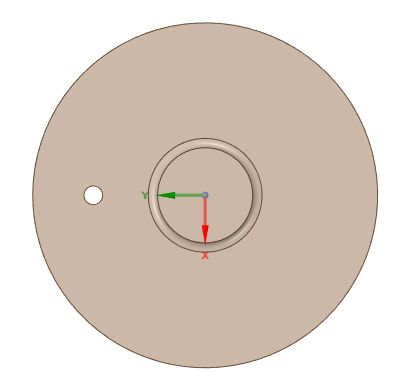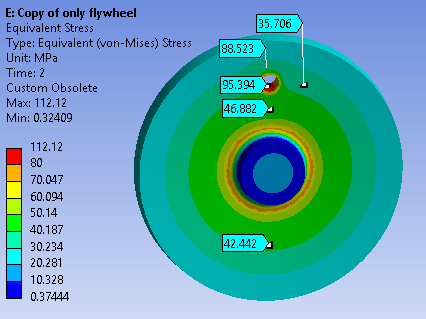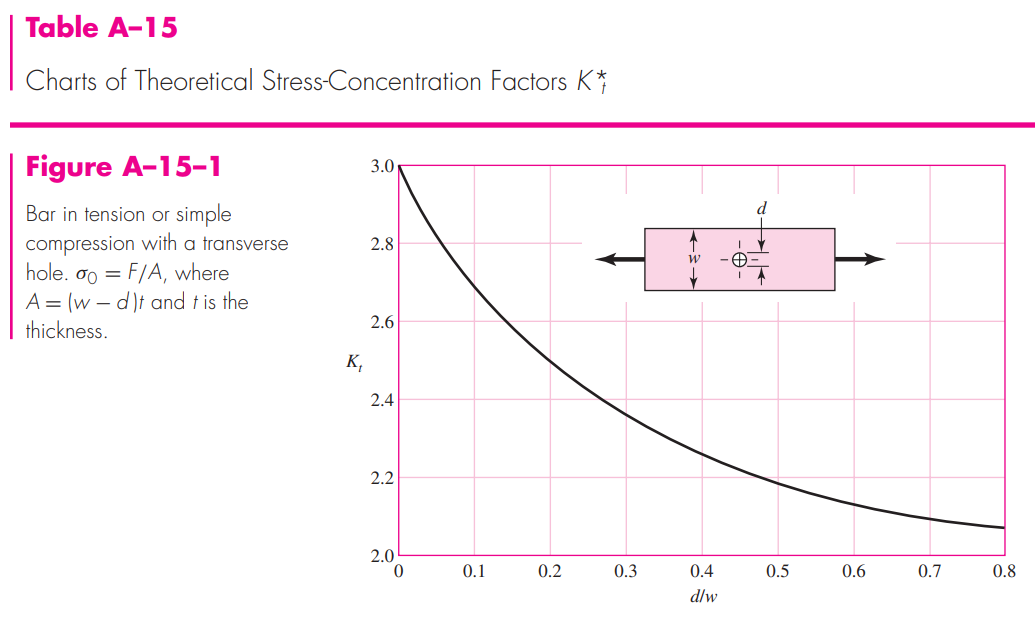## General Mechanical

•arvindp
Subscriber
Hi nI am simulating a large rotating flywheel which has a hole in it, that causes stress concentration. I have simulated the condition and obtained results. Now the issue is, I want to verify it analytically. What formulae do I use? Can someone explain the concept? or can anyone suggest me some good literature to understand the analytical calculations?n
•1shan
Ansys Employee
nThere are certain things ANSYS employee can't do on the forum and unfortunately I can't point you to a particular article. Maybe some other forum members could pitch in. Please let us know if you find any difficulty while simulating the problem in ANSYS.nAll the best.nIshann
•peteroznewman
Subscriber
Though I don't work at ANSYS, I think I understand the constraints on employees. You are free to point to any public article and any part of the ANSYS Help system. nhttps://forum.ansys.com/discussion/23093/why-ansys-employees-dont-download-attachmentsnIt would help if you reply with images of the disk and the hole with dimensions and other details like the rotational velocity.nn
•arvindp
Subscriber
the hole diameter is 100 mm, the disk diameter is 1950 mm. the distance of the hole from the disk center does not need to be exact. assume it is somewhere midway. mass of the flywheel is 4.5 tons. rotational speed being 1380 rpm.nyou can see that there are high stresses on the top and bottom of the hole ID. how is it happening and why? can we calculate this analytically?n
•peteroznewman
Subscriber
nShigley shows the stress concentration factor of a hole in a plate under uniaxial tension. You have a similar stress concentration. Why do you need to calculate it analytically?n•arvindp
Subscriber
thank you for pointing out this concept. i had referred to it earlier but i did not know how to proceed with it (i.e. what force to apply, how to exactly calculate the centrifugal force, etc.) because i tried doing it analytically but could not match the simulation results. hence, i am here.nnand to answer your question, we have a lot of such applications and simulating each one of them is time consuming. in fact the snaps are a simplified version of our problem. i am not allowed to share the actual problem which includes non linearity. but lets first solve this. the solution to this small question here will lead me to the solution to the bigger problem i have to solve.n
•peteroznewman
Subscriber
nIf you often have a disk with a hole in it, but the parameters change, such as the disk diameter, the hole diameter the radius to the center of the hole, the rotational velocity etc. you should set up a parametric model. That way you just type in those four values into the Parameter Set Table and hit Update All Design Points and you will get the stress out. You could make this a shell model so the solve time is very short. You can get answers for multiple rows of designs at one time.n
•YasserSelima
Subscriber
The hole in the disk, causes a force similar to added mass. ni.e The small mass imbalance would cause an oscillating force = m * omega^2 * e , pointing in the radial direction. ... m takes a positive sign for added mass and -ve sign for a subtracted mass.nn
•arvindp
Subscriber
Thank you, nbut i notice this stress on symmetric holes too, therefore it occurs in spite of imbalance n
•YasserSelima
Subscriber
nBalancing the mass, does not mean we removed the force. We just added a similar force on the other side to balance the forces on the shaft.n